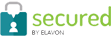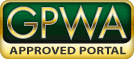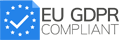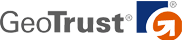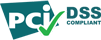Betting Tools > Odds Converter

# Odds Calculator - Convert Betting Odds & Calculate Payout

Betting odds calculator allows you to insert your odds and automatically convert them to American, Decimal, and Fractional odds. It also calculates the implied probability of the bet and the profit if the bet wins. The default wager is set at \$100.

Error
More betting tools: Parlay Calculator

## Understanding betting odds

The way sports betting odds are presented can differ between American, Fractional, and Decimal. While they all mean the same thing, understanding how they work with your wager can be tricky. Use our betting odds and moneyline calculator tool above to convert these odds and learn more about them below.

## American odds explained

American odds are presented in hundreds and thousands and are defined by the positive (+) or negative (-) value assigned. An easy way to think about American odds calculation is to think of them as currency exchanges in \$100 increments.

The most common American odds you’ll see is -110 and is often seen as the juice/vig on points spreads and Over/Under Totals

• 110 odds require a \$110 wager to win \$100.

Bets with higher implied probability are given a higher negative value. For example, a bet placed at -400 odds would require you to wager \$400 to profit \$100.

Bets with lower implied probability are given a positive value. For example, a bet placed at +400 odds would profit \$400 on a \$100 wager. The positive value indicates that the odds are “plus money” and return more profit than the amount risked on the bet.

## Decimal odds explained

Decimal odds are the preferred variation for most countries outside of the U.S., including Europe, which is why they are often referred to as “European odds”.

Instead of using positive and negative values or fractional equations, decimal odds display a simple value that you multiply your bet amount by to calculate your profit (money won) and overall return (profit and original risk amount).

For example, a \$100 bet made at decimal odds of 3.00 would return \$300 (\$100 x 3.00): \$200 in profit and the original \$100 amount risked. A \$100 bet made at decimal odds of 1.50 would return \$150: \$50 in profit and the original \$100 amount risked.

## Fractional odds explained

Fractional odds are most common in horse racing and futures markets. They’re essentially simple math: Dividing one number by another and then taking that sum and multiplying it by your bet amount.

If the larger number is being divided by the smaller number, such as 3/1, then the odds will pay out more than the original bet risked. But if the smaller number is divided by, the larger number, such as 1/3, then the odds will pay out less than the original bet risked.

For example, odds of 3/1 will pay three times (300 percent) the amount risked, so a bet of \$100 would profit \$300 if it wins. Odds of 1/3 will pay only 33.33 percent of the amount risked, so a bet of \$100 would profit \$33.33 if it should win. Odds of 1/1 will pay the same amount risked, so a bet of \$100 would profit \$100 if it should win.

Some fractional odds will see the dividend divided by a value greater than one – ie: 7/2 or 11/4 but is still calculated the same, multiplying the sum by the amount risked. Odds of 7/2 will pay 3.5 times the bet amount, so for example, a \$100 bet placed on 7/2 fractional odds will return \$350 in profit. A \$100 bet placed on 2/7 odds will return \$28.57 in profit.

## Implied probability explained

Covers’ odds converter allows you to enter any of these odds variations and get them instantly translated into your preferred display, while also calculating the implied probability of those odds winning outright.

For example, a bet placed at +200 in American odds (2/1 in fractional or 3.00 in decimal) has a 33.33 percent implied winning probability. A bet placed at -200 in American odds (1/2 in fractional or 1.50 in decimal) has a 66.67 percent implied winning probability.

You can also define your odds conversion to a specific bet amount to see your expected profit if that wager should win. Odds with lower implied probability have larger risk and therefore pay out more compared to odds with higher implied probability and lower risk.

For example, a \$100 bet at -200 in American odds (1/2 in fractional or 1.50 in decimal) with a 66.67 percent implied probability has the potential to profit \$50 should it win (not total return, which would include your original bet amount). A \$100 bet at +200 in American odds (2/1 in fractional or 3.00 in decimal) with a 33.33 percent implied probability has the potential to profit \$200 should it win.

## FAQs

The Covers community has
549,913
Covers is verified safe by: